# DAV Class 5 Maths Chapter 6 Worksheet 4 Solutions

The DAV Maths Book Class 5 Solutions and DAV Class 5 Maths Chapter 6 Worksheet 4 Solutions of Addition and Subtraction of Decimal Numbers offer comprehensive answers to textbook questions.

## DAV Class 5 Maths Ch 6 Worksheet 4 Solutions

Question 1.
Subtract.
(a)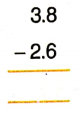Solution: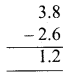(b)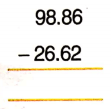Solution: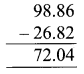(c)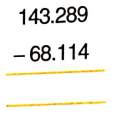Solution:(d)Solution: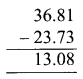(e)Solution:(f)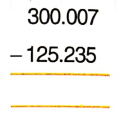Solution:Question 2.
Arrange in columns and Subtract.
(a) 6.3 from 9.5
Solution:
Convert into like decimals and then subtract.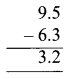(b) 71.86 from 95.97
Solution: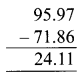(c) 315.28 from 486.195
Solution:(d) 19.378 from 26.4
Solution: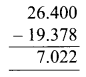(e) 45.6 from 55.352
Solution: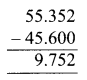(f) 22.05 from 319.019
Solution:(g) 71.084 from 90.04
Solution: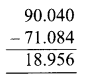(h) 174.5 from 200.17
Solution:DAV Class 5 Maths Chapter 6 Worksheet 4 Notes

We can subtract decimal numbers in the same way as we subtract whole numbers.

Example 1.
Subtract 18.73 from 29.75Arrange the digits in the place value columns.
Put decimal point one below the other.
Subtract subtrahend and minuend.Example 2.
Subtract 46.13 from 59.41359.413 – 46.130
Convert into like decimals
Arrange in column.
The decimal point below the other.
Subtract subtracted and minuend.